# SSAT Upper Level Math : Properties of Exponents

## Example Questions

← Previous 1 3 4 5 6 7 8 9 12 13

### Example Question #1 : How To Find The Properties Of An Exponent

Evaluate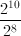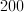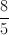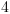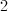Explanation:

If you divide two exponential expressions with the same base, you can simply subtract the exponents.  Here, both the top and the bottom have a base of 2 raised to a power.

So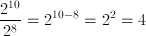### Example Question #1 : Generate Equivalent Numerical Expressions: Ccss.Math.Content.8.Ee.A.1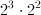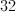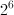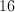Explanation:

Since the two expressions have the same base, we just add the exponents.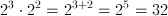### Example Question #11 : Generate Equivalent Numerical Expressions: Ccss.Math.Content.8.Ee.A.1

Evaluate: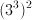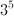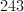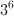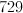Explanation:

A power raised to a power indicates that you multiply the two powers.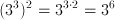### Example Question #1 : How To Find The Properties Of An Exponent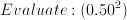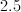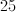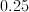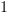Explanation:

We can either write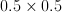, or we can convert this to a fraction and write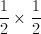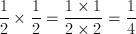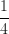in decimal form is 0.25.

### Example Question #1 : Properties Of Exponents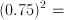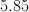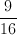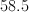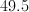Explanation:

Convert .75 to a fraction.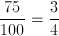.

Now multiply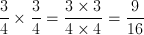### Example Question #4 : How To Find The Properties Of An Exponent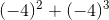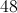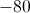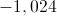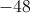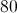Explanation: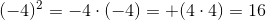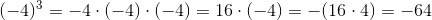Therefore,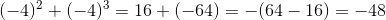### Example Question #1 : How To Find The Properties Of An Exponent

Express 0.00000000000097 in scientific notation.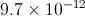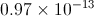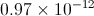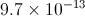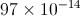Explanation:

To rewrite a very small number in scientific notation:

Write the number.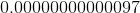Move the decimal point right as many places as needed until it follows the first nonzero digit, which here is the nine. Count the number of places it is moved - here it will be thirteen places.

The number formed is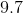, which will be placed in front;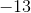, the negative of the number of places counted, will be the exponent. The number, in scientific notation, will be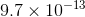.

### Example Question #1 : How To Find The Properties Of An Exponent

Evaluate: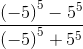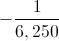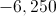The quantity is undefined.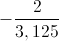The quantity is undefined.

Explanation: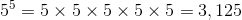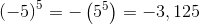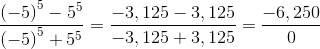This is an undefined quantity.

### Example Question #2 : How To Find The Properties Of An Exponent

Evaluate: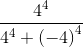The quantity is undefined.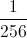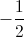Explanation: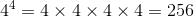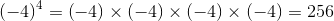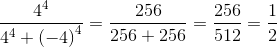### Example Question #12 : Expressions & Equations

Evaluate: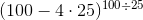The expression is undefined.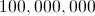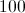Explanation: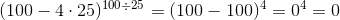as 0 taken to any positive power is equal to 0.

← Previous 1 3 4 5 6 7 8 9 12 13

### All SSAT Upper Level Math Resources